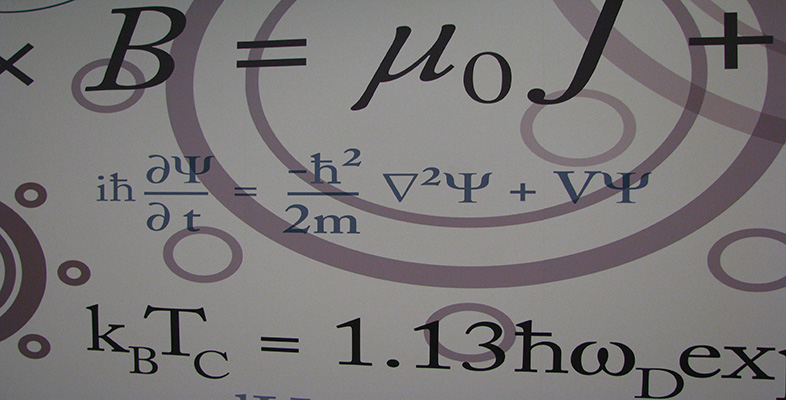Number systems

This free course is available to start right now. Review the full course description and key learning outcomes and create an account and enrol if you want a free statement of participation.

Free course

3.2 Congruence

The Division Algorithm tells us that, when we divide any integer by a positive integer n, the set of possible remainders is {0, 1, 2, …, n − 1}. Integers which differ by a multiple of n have the same remainder on division by n and are, in this sense, ‘the same’ as each other. We now introduce some notation and terminology for this idea of ‘sameness’, which is known as congruence.

Definitions

Let n be a positive integer. Two integers a and b are congruent modulo n if ab is a multiple of n; that is, if a and b have the same remainder on division by n.

In symbols we write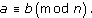(We say ‘a is congruent to b modulo n’.)

Such a statement is called a congruence, and n is called the modulus of the congruence.

Note: This is a different meaning for the word ‘modulus’ from others you may have met. It is important to interpret technical terms according to their context.

Example 10

Which of the following congruences are true, and which are false?

• (a)  27 ≡ 5 (mod 11)

• (b)  14 ≡ −6 (mod 3)

• (c)  343 ≡ 207 (mod 68)

• (d)  1 ≡ −1 (mod 2)

Solution

• (a)  27 − 5 = 22, which is a multiple of 11, so this congruence is true.

• Alternatively, we could note that 27 = 2 ×11 + 5 and 5 = 0 ×11 + 5, so 27 and 5 both have remainder 5 on division by 11.

• (b)  14 − (−6) = 20, which is not a multiple of 3, so this congruence is false.

• Alternatively, 14 = 4 × 3 + 2 and −6 = (−2) × 3 + 0, so 14 has remainder 2 on division by 3, but −6 has remainder 0.

• (c)  343 − 207 = 136 = 2 × 68, so this congruence is true.

• Alternatively, 343 = 5 × 68 + 3 and 207 = 3 ×68 + 3, so 343 and 207 both have remainder 3 on division by 68.

• (d)  1 − (−1) = 2, so this congruence is true.

• Both 1 and −1 have remainder 1 on division by 2.

It is often simplest to check a congruence ab (mod n) by considering the difference ab.

Exercise 39

Find the remainder on division by 17 of each of the numbers 25, 53, −15, 3 and 127, and state any congruences modulo 17 that exist between these numbers.

Solution

We have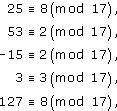so the remainders are 8, 2, 2, 3 and 8, respectively. So 25 ≡ 127 (mod 17) and 53 ≡ −15 (mod 17).

We shall need to use some properties of congruences in the following sections, and we state these properties here.

Theorem 5 Properties of congruences

Let n and k be positive integers, and let a, b, c, d be integers. Then

• (a)  aa (mod n);

• (b)  if ab (mod n), then ba (mod n);

• (c)  if ab (mod n) and bc (mod n), then ac (mod n);

• (d)  if ab (mod n) and cd (mod n), then a + cb + d (mod n);

• (e)  if ab (mod n) and cd (mod n), then acbd (mod n);

• (f)  if ab (mod n), then akbk (mod n).

Although this may seem a long list, these properties are quite simple.

Proof

We prove properties (a)–(d) here and ask you to prove properties (e) and (f) in Exercise 40.

• (a)  aa = 0 = 0 × n, so aa (mod n).

• (b)  Suppose that ab (mod n). Then ab = kn for some integer k.

•     Hence ba = (−k)n, so ba (mod n).

• (c)  Suppose that ab (mod n) and bc (mod n). Then ab = kn and bc = ln for some integers k and l. Hence

•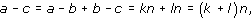• so ac (mod n).

• (d)  If ab (mod n) and cd (mod n), then ab = kn and cd = ln for some integers k and l. Hence a = b + kn and c = d + ln, so

•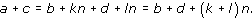• Hence (a + c) − (b + d) = (k + l)n, so a + cb + d (mod n).

Exercise 40

• (a)  Use a similar method to that used in part (d) of the above proof to prove property (e) of Theorem 5.

• (b)  Use property (e) and mathematical induction to prove property (f) of Theorem 5.

Solution

• (a)  Suppose that ab (mod n) and cd (mod n). Then a = b + kn and c = d + ln for some integers k and l.

• Hence

•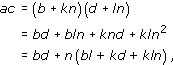• so acbd = (bl + kd + kln)n, so acbd (mod n).

• (b)  Suppose that ab (mod n) and let P(k) be the statement akbk (mod n).

• Then P(1) is true, since we know that ab (mod n). Suppose that r ≥ 1 and P(r) is true, that is, arbr (mod n). Then, by property (e), since arbr and ab (mod n), we have ar × abr × b (mod n), that is,

•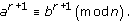• Hence P(r) true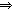P(r + 1) true, so by mathematical induction P(k) is true for all positive integers k.

The properties in Theorem 5 are particularly useful when finding the remainder of a large integer on division by another integer.

Example 11

• (a)  Find the remainders of both 2375 and 5421 on division by 22.

• (b)  Find the remainder of 2375 × 5421 on division by 22.

• (c)  Find the remainder of (2375)15 on division by 22.

(2375)15 is too large to fit into the memory of most computers, so there is a real advantage in this method.

Solution

• (a)  Using property (c), we can add or subtract any convenient multiples of 22, and make a list of congruences. We have

•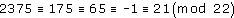• (here we have subtracted 2200, then 110, then 66; then added 22)

• and

•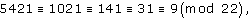• (here we have subtracted 4400, then 880, then 110, then 22)

• so 2375 has remainder 21 on division by 22, and 5421 has remainder 9 on division by 22.

• (b)  Using property (e), we obtain

•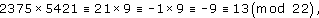• so 2375 × 5421 has remainder 13 on division by 22.

• (c)  Using property (f), we obtain

•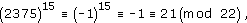• so (2375)15 has remainder 21 on division by 22.

M208_6